# Diodes derive logarithms and exponentials to optically sense AC/DC current

## Texas Instruments LM324

Stephen Woodward

EDN

In theory, the design of current sensing circuitry, typically based on simple measurement of the IR voltage developed across a resistor placed in series with the current to be sensed, looks like an easy task. In theory. But in practice, complicating factors sometimes combine to make it a much more interesting exercise.

For example, in an effort to minimize efficiency and power lost in the current sampling resistor, its resistance is typically limited to milliohms, the IR voltage developed to millivolts, and the resulting diminutive signal may ride on and need to be extracted from the common mode of a supply rail carrying 10s (sometimes 100s) of volts in the company of large noise components. These design challenges are reflected in the development of many innovative topologies and application specific devices (52 of the latter from Analog Devices alone!).

This Design Idea addresses this classic problem from a different angle. Using only generic components, it implements an optically-isolated (and therefore common-mode immune) current sense topology compatible with DC sources of either polarity, and AC too. The resulting circuit might fairly be called a universal current sensor that’s also cheap, as it incorporates no part costing more than \$1. Here’s how it works (Figure 1).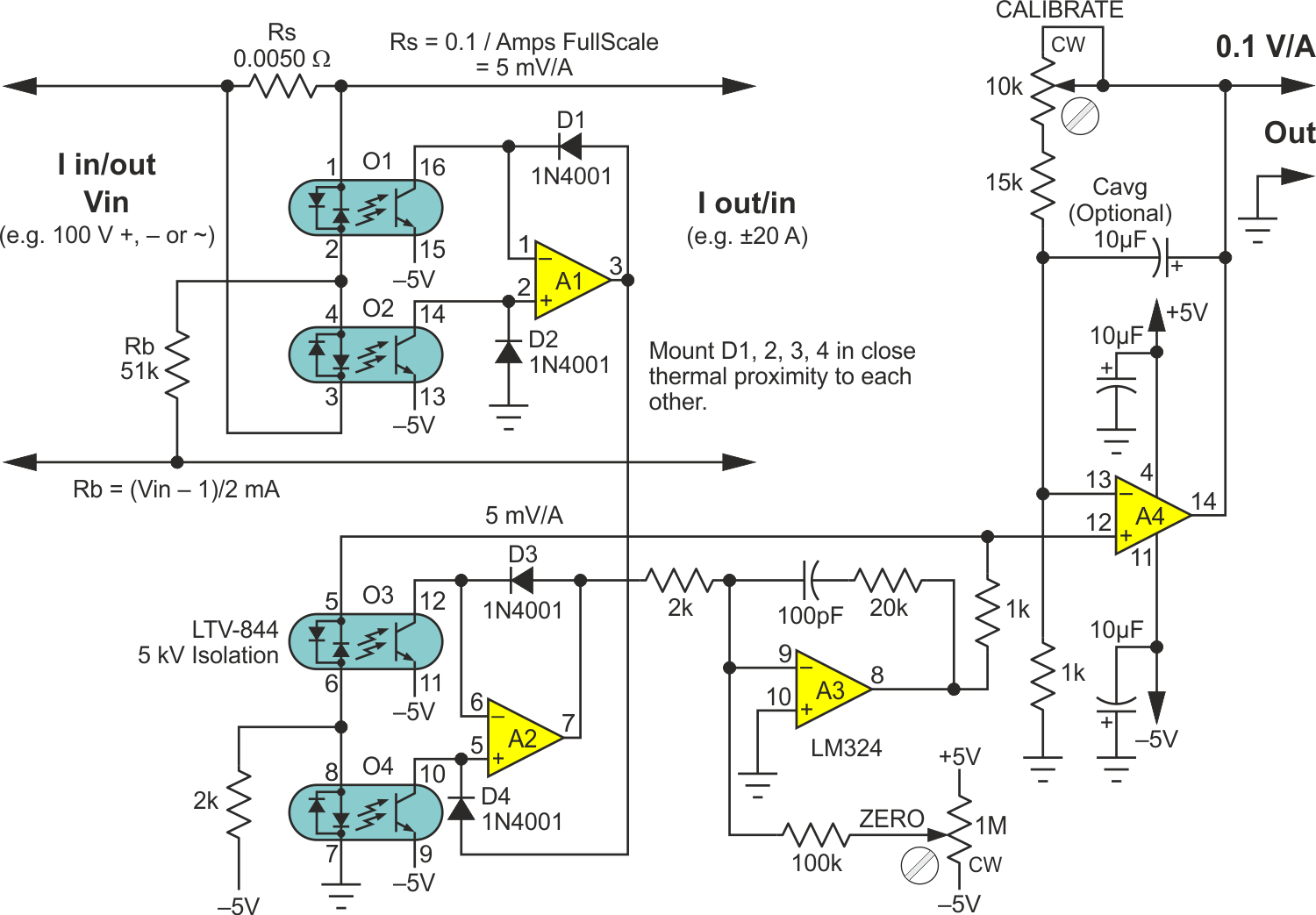Figure 1. The optically-isolated bipolar current sense schematic for this Design Idea.

Referring to the schematic, shunt resistor Rs fills the role of the usual current sampling resistance, setting a measurement full-scale factor of Is = 0.1/Rs = 20 Amperes in this example with Rs = 5 milliohms. But most any full scale current can be selected by choosing a suitable resistor. Voltage Vs developed by Rs is sampled by series connected LEDs of optocouplers O1 and O2 according to a variant of the classical diode equation (graphically gleaned from manufacturers’ datasheet I/V curves):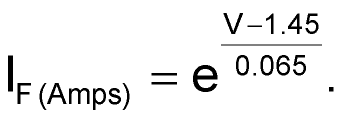LEDs, despite their remarkable ability to emit light, are still just diodes under the skin and their log/exponential functionality is usefully accurate so long as IF is limited to ~0.0001th to 0.1th of their max rated current. A similar criterion for useful log/exponential computation applies to the 1N4001s also used here – likewise obtained.

Back-to-back series connection of the O1 and O2 LEDs subtracts and cancels out the diode equation fixed 1.45 V term, leaving the bias current Ib (nominally 2 mA) supplied by Rb to be split in the ratio: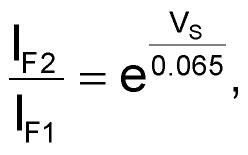which varies from 1.00 at Vs = 0 (zero current) to: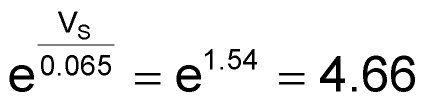at Vs = 100 mV (20 A × 0.005 Ohms).

Note that the ratio of the split is independent of the precise magnitude of Ib, leaving the accuracy of ratio-based current measurement largely immune to Ib and therefore supply voltage variation. Note also the ability of the ratio computation to respond to current reversal, e.g.: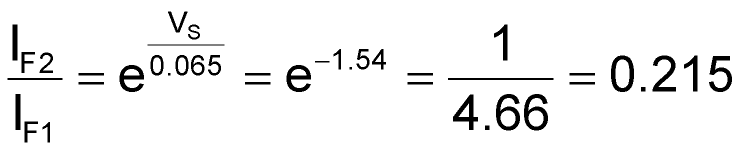at Vs = −100 mV

While these numbers will change with swings in temperature, it’s irrelevant to accuracy so long as temperatures of the LEDs track each other in the package they share. Moreover, because each LTV-844 emitter actually comprises a pair of identical LEDs connected antiparallel, reversal of applied voltage simply switches emission from one LED in the pair to the other, leaving the current sensing function affected. This provides the bipolar and AC capabilities mentioned earlier.

O1/O2 LED emission then occurs in proportion to the IF2/ IF1 ratio, causing a similar ratio in coupled photo transistor collector currents (CTR ~80% @ IF = 1 mA), which are input to D1 and D2, generating forward voltages according to the 1N4001 version of the diode equation: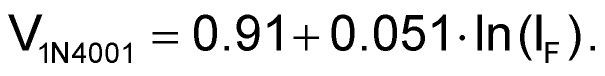Differential V1N4001 summation results in: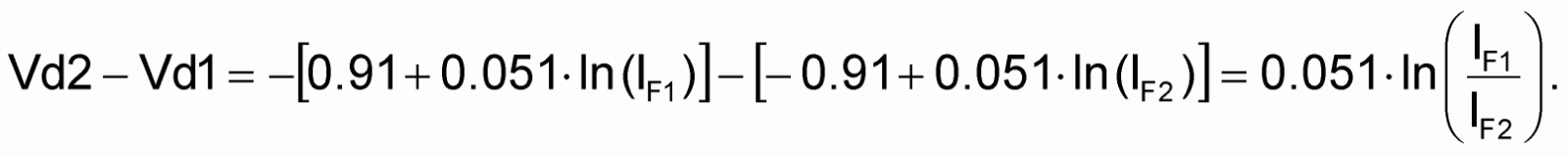This signal is then subtracted from an identical log-ratio calculation by D3, D4, and A2 taken from reference optos O3 and O4, controlled by feedback amplifier A3. The net result is that, in nulling A2’s output, A3 implicitly calculates a drive signal for O3/O4 that accurately and linearly mirrors the current sense signal at O1/O2. This signal is scaled by A4 to become the final current sense output, with an optional Cavg integrating capacitor to filter the full-wave rectified waveform that results from sensing AC. Without Cavg, bandwidth of the sensor is roughly 50 kHz. With Cavg, roughly 0.8 Hz (RC = 200 ms) for <1% ripple at 60 Hz.

Output signal polarity reflects whether current passes forward from lN to OUT ports (positive), or backwards from OUT to IN (negative). This is useful in battery-condition monitoring, in which simple integration of the output signal will reflect net battery discharge vs charge.

Accommodation and correction of production variation in the active devices is provided by zero (offset) and calibrate (gain) trimmers. Optimum cancellation of temperature sensitivity of diode equation terms is accomplished by thermal proximity in the mounting of log ratio computing diodes D1 – D4 and sharing of a common package by optos O1 – O4. This is simplified by the fact that the only property of the D1 – D4 analog computation that really matters is its sign.

The resulting current sensor is inexpensive, versatile, and robust, being able to shrug off current transients of more than 20× full scale (Rs thermal capacity being the limiting factor), and large common mode differentials. (The LTV-844 being rated for 5 kV!).

EDN